Next: Application to Mineral Extraction Up: The Second Law of Previous: The Second Law of

# Theory

The entropy per mole of an n-component ideal mixture is given by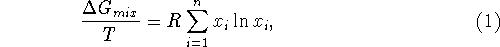where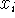is the mole fraction of the ith constituent. If there are only two constituents, we have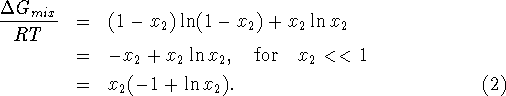If the mixture has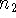moles of the second constituent, we get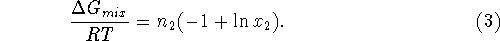The energy required to separate the mixture is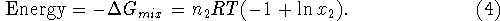For those who didn't follow the derivation, of (4), we summarize. R is the universal gas constant 8.314 joules per mole per degree Kelvin, T is the absolute temperature at which the separation takes place and x is the mole fraction in the mixture of the substance being extracted.

John McCarthy
Thu Aug 21 09:12:21 PDT 1997